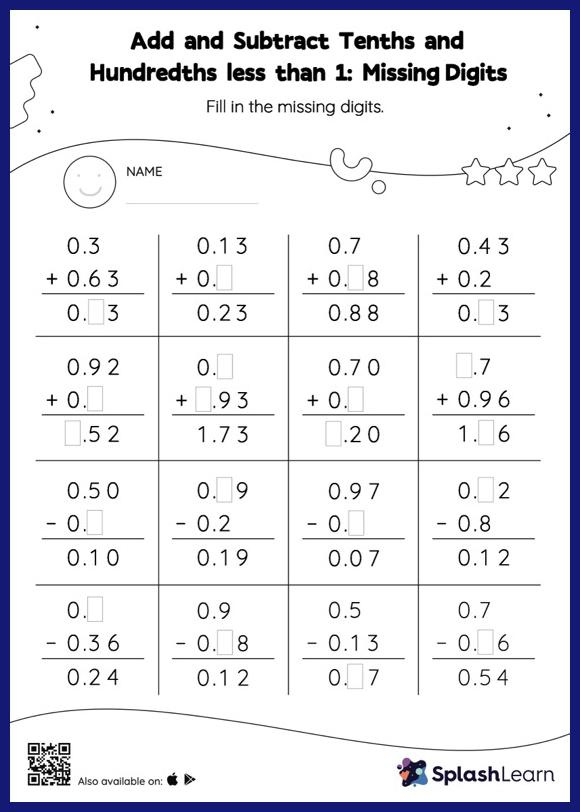# Add and Subtract Tenths and Hundredths less than 1: Missing Digits Worksheet

Home > Add and Subtract Tenths and Hundredths less than 1: Missing DigitsThis worksheet consists of a set of questions on addition and subtraction where students are required to add and subtract tenths and hundredths less than 1. When adding or subtracting decimals on this worksheet, students align the decimal points and use zero as a placeholder. Then to find the missing number in the add and subtract tenths and hundredths less than 1 worksheet, students then apply the relationship between addition and subtraction.